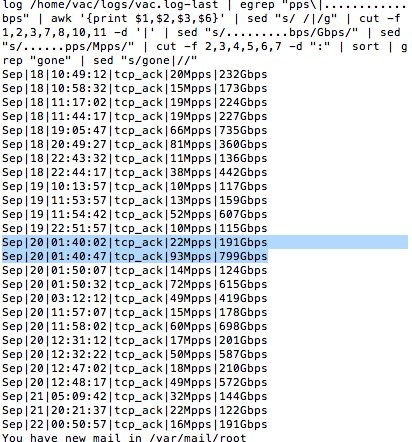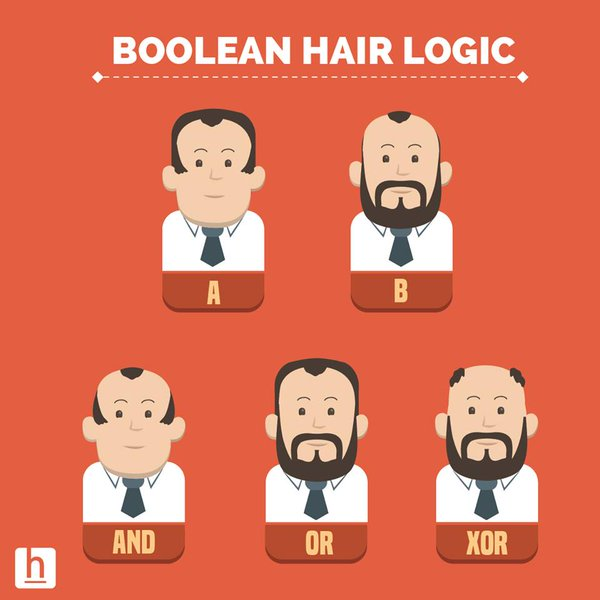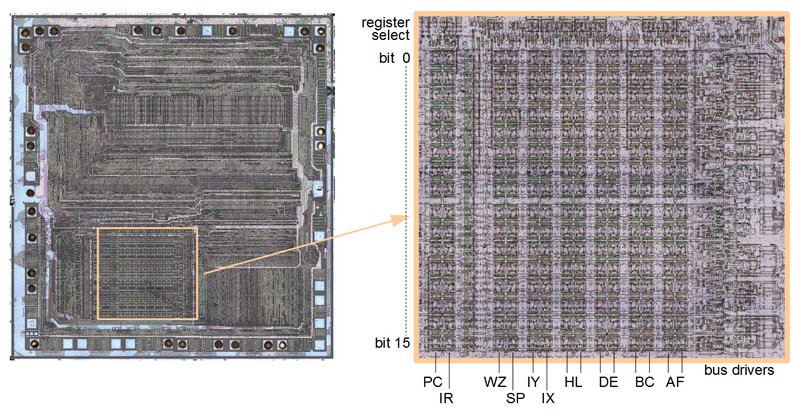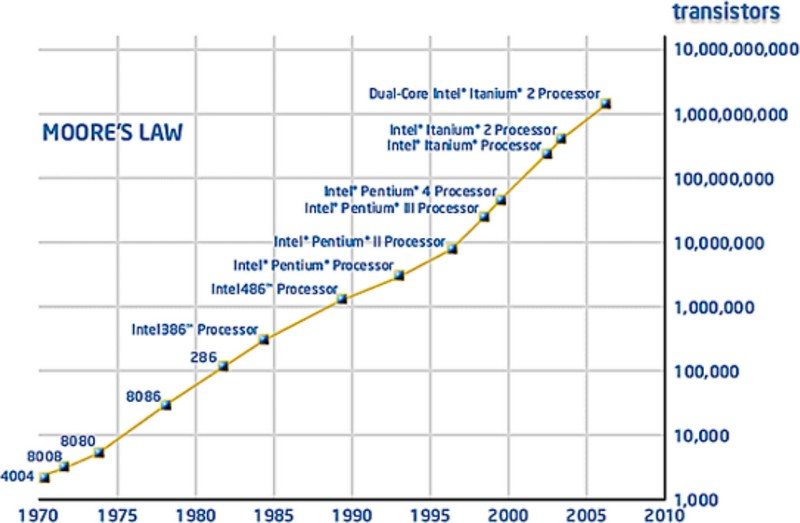# Ordinal Data - Comparison Operator (Order, Equality)

A comparison operator is a class of operators that model:

A comparison operator test the order relationship between two operands and returns a Boolean.

The result of a comparison is a Boolean

## Usage

### Sort

Comparison Operator are tiddly coupled:

in order to sort data.

### Condition / Filter

Comparison Operator are used in predicate (condition) in order to filter

## Properties

### Order of precedence

order of precedence - All comparison operators have the same priority, which is lower than that of all operator mathematical operators

### Chain

Comparisons may be chained.

For example, a < b == c tests whether:

• a < b
• and moreover b == c

### Combination

Comparisons may be combined using the Boolean operators and and or to from a predicate (boolean expression)

## Relation

Comparison operator models two kind of relation:

Binary relation are used in many branches of mathematics to model this relation. See Logical Data Modeling - Binary Relation.

### Order

Order relation, including strict orders:

• greater than or equal to
• less than or equal to
• divides (evenly)
• is a subset of
Operation Description
< strictly less than
<= less than or equal
> strictly greater than
>= greater than or equal
in check whether a value occurs in a list
not in check whether a value does not occur in a list

### Equivalence

The equal operators model an equivalence relationship

## Documentation / Reference

Recommended PagesBash - Conditional Expression

A predicate or boolean expression is known in Bash as a Conditional Expression. It is a command that returns an exit status of 0 or 1. The conditional expression is part of the compound expression. ...Bash - Integer

This article is the management of integer in bash. See See The ordinal comparison operator in Bash are: Double Bracket operator Single Bracket Operator Example -lt -lt [[ 8 -lt 9 ]] && echo "8 is less than 9"...Boolean - (Logical) Operator (OR, AND, XOR, )

Boolean operator manipultes truthy and falsy values that can come from: a Boolean value the interpretation of a non-boolean value (ie javascript (truthy/falsy)) or the result of a comparison expression....Boolean - Predicate (Boolean Expression, Condition)

A Predicate is an boolean expression that evaluates to: TRUE, FALSE, or UNKNOWN. A predicate acts as a filter that filters a certain number of element from a collection (set) where: ...CPU Register - Control registers

Control registers: instruction pointer register (32-bit) flags register (32-bit) to perform: comparisons mathematical calculations flow implementation by changing the status of flags (conditional...Collation - String comparison

Collation is a general term for the process and function of determining the sorting order of strings of characters (or in other term, how a strings comparison is performed) Collation implementations must...Computer Architecture - Arithmetic logic unit (ALU)

The ALU is a component of a CPU. It is a device that executes all arithmetic and logical operations. In most arithmetic logic unit (ALU) hardware, we find a fixed-precision arithmetic found , which...Data Type - Boolean

A Boolean is a primitive data type and may be implemented with: only two state: true or false 1 or 0 and an optional third one It's therefore almost always implemented on a computer level with...Function - Binary Function/Operation

A binary operation is an scalar operation with two arguments (arity of two) that produces one value. They are creating a binary relation. the addition operator, the multiplication operator ...Function - Order

Ordering is the process of finding the position of an element of ordinal data type in a sequence. Order and Equivalence are a special kind of binary relation (ie relation between two entities) With the...